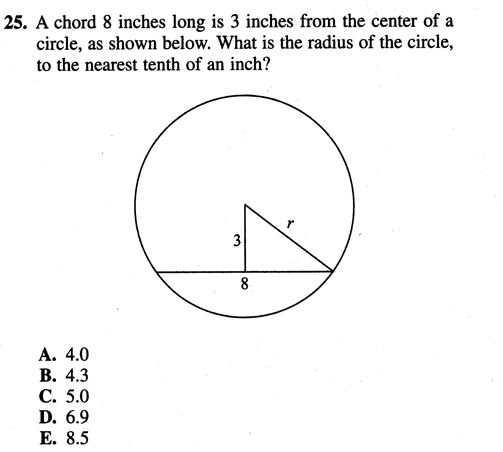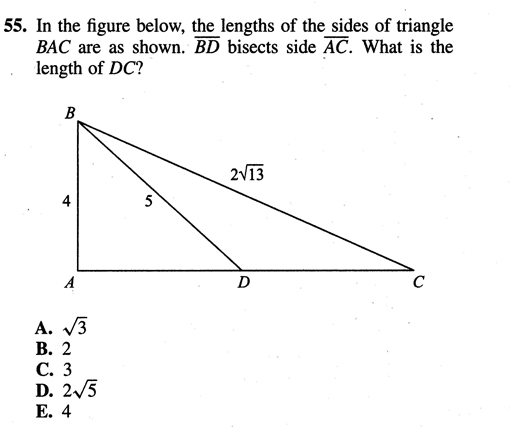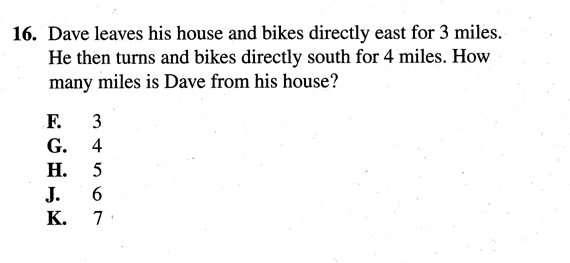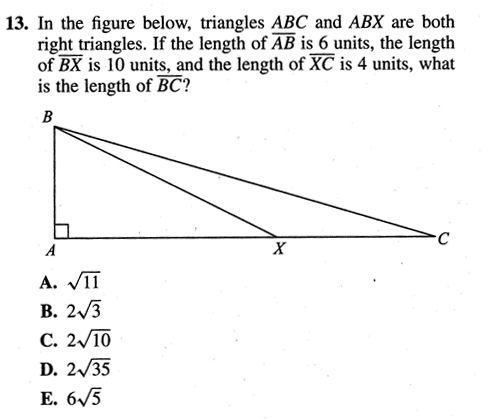Imagination is more important than knowledge... Albert Einstein

Guess is more important than calculation --- Knowhowacademy.com

Length - Pythagorean Theorem

For ACT
Number of Total Problems: 28.
FOR PRINT ::: (Book)

 Problem Num : 1 Type: None Section:Length Theme:None Adjustment# : 10 Difficulty: 1Category Pythagorean Theorem
Analysis

 Problem Num : 2 Type: None Section:Length Theme:None Adjustment# : 13 Difficulty: 1Category Pythagorean Theorem
Analysis

 Problem Num : 3 Type: None Section:Length Theme:None Adjustment# : 14 Difficulty: 1Category Pythagorean Theorem
Analysis

 Problem Num : 4 Type: None Section:Length Theme:None Adjustment# : 15 Difficulty: 1Category Pythagorean Theorem
Analysis Funny question!

 Problem Num : 5 Type: None Section:Length Theme:None Adjustment# : 15 Difficulty: 1Category Pythagorean Theorem
Analysis can you see..3, 4, 5? This is the hide and seek question.

 Problem Num : 6 Type: None Section:Length Theme:None Adjustment# : 16 Difficulty: 1Category Pythagorean Theorem
Analysis 3-4-? Hide and Seek!

 Problem Num : 7 Type: None Section:Length Theme:None Adjustment# : 17 Difficulty: 1Category Pythagorean Theorem
Analysis

 Problem Num : 8 Type: None Section:Length Theme:None Adjustment# : 18 Difficulty: 2Category Pythagorean Theorem
Analysis

 Problem Num : 9 Type: None Section:Length Theme:None Adjustment# : 18 Difficulty: 1Category Pythagorean Theorem
Analysis

 Problem Num : 10 Type: None Section:Length Theme:None Adjustment# : 19 Difficulty: 2Category Pythagorean Theorem
Analysis